# Digging Deeper into … Addition and Subtraction (3rd to 6th class)

## Digging Deeper into … Addition and Subtraction (3rd to 6th class)

Category : Uncategorized

For practical suggestions for families, and links to useful digital resources, to support children learning about the topic of addition and subtraction, please check out the following post: Dear Family, your Operation Maths Guide to Addition and Subtraction

Addition and Subtraction is always the first operation’s chapter in Operation Maths 3-6, and it is always a double chapter i.e. it is structured to be covered over 10 days/two school weeks. In Operation Maths 3-5 there is also a second Addition and Subtraction chapter (this time only a single i.e. one week chapter) in the second half of the school year to revise and re-focus on specific strategies that can be used.

### Relationship between addition and subtraction

In contrast to traditional maths schemes, which often have separate chapters for each operation, Operation Maths instead teaches addition and subtraction together, as related concepts. Teaching the operations in this way will encourage the children to begin to recognise the relationships between addition and subtraction, and indeed all the operations. Thus, the initial activities in the Discovery Book, require the children to reflect on their understanding of the concepts and to compare and contrast them.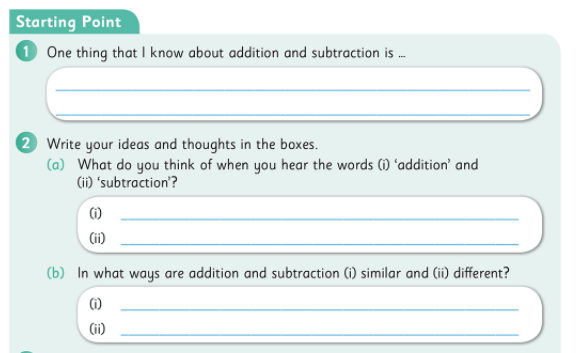In particular, the children are enabled to understand addition and subtraction as being the inverse of each other and are encouraged to use the inverse operation to check calculations.### Looking at the bigger picture

Children can often have tunnel vision (or column vision) regarding addition and subtraction calculations: they “do” the units, then the tens, then the hundreds without really looking at the whole numbers or the processes involved.One way in which you can encourage the children to look at and understand these operations better is by using a Concrete–pictorial–abstract (CPA) approach. This means the children will be moving from experiences with the familiar base ten concrete materials (e.g. straws, base ten blocks, money, the Operation Maths place value discs, pictured above) to pictorial activities (e.g. where the children draw representations of the numbers using pictures of the concrete materials or use empty number lines, bar models, etc.) and finally to abstract exercises, where the focus is primarily on numbers and/or digits.

When exchanging tens and units or tens and hundreds, reinforce that a ten is also the same as 10 units, and that a hundred is the same as 10 tens and 100 units.
The use of non-canonical arrangements of numbers (e.g. representing 245 as 2H 3T 15U or 1H 14T 5U), as mentioned in Place Value, can also be very useful to children as they develop their ability to visualise the regrouping/renaming process. The Operation Maths Place Value eManipulative, accessible on edcolearning.ie,  is an excellent way to illustrate this and explore the operations in a visual way. Worth noting also, is that the Operation Maths Place Value eManipulative and place value discs provide the only means to concretely or pictorially represent base ten materials to five whole number places (no other interactive tool is available on the internet to do this); a fact which will be of particular value to teachers of 5th and 6th classes who didn’t have a way to concretely/visually represent numbers to ten thousands prior to the inception of Operation Maths.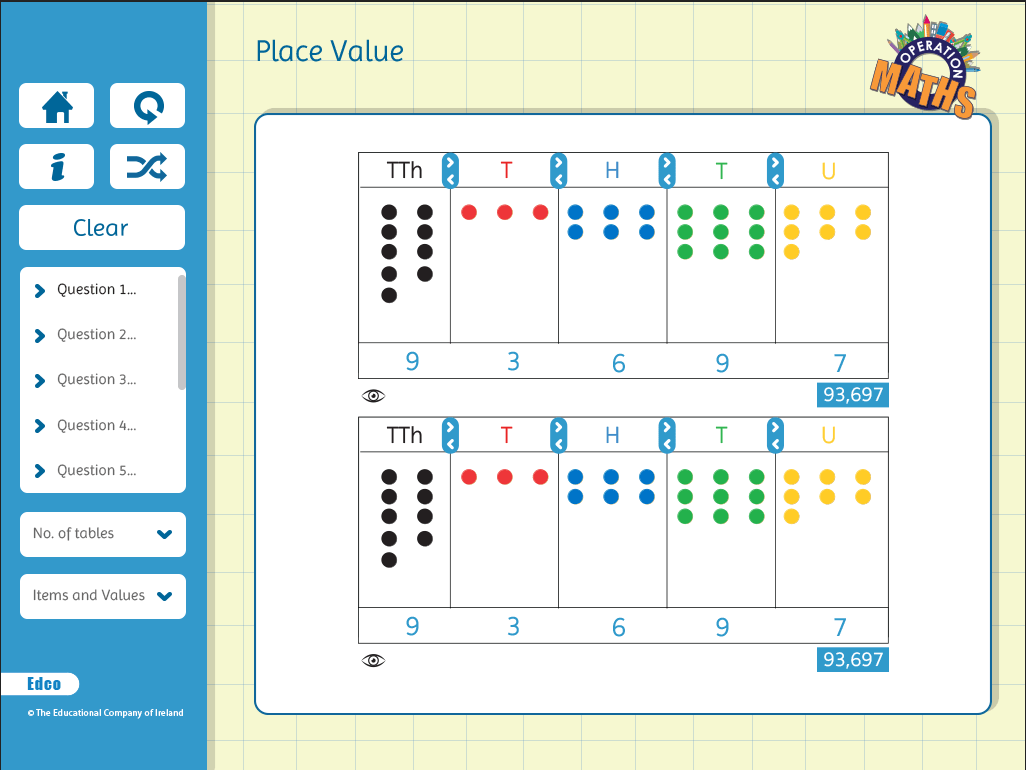### Mental strategies are as important as written methods

The traditional, written algorithms for addition and subtraction, i.e. the column methods, are important aspects of these operations. However, in real-life maths, mental calculations are often more relevant than written methods. Also, as mentioned previously, children can often have tunnel vision (or column vision) regarding addition and subtraction calculations; they ‘do’ the units, then the tens, then the hundreds, etc., without really looking at the entire numbers or the processes involved. Therefore, while the column method for addition and subtraction is a main part of this topic, equally important is the development of mental calculation skills, using such strategies as those outlined on this page from Operation Maths 6 (below)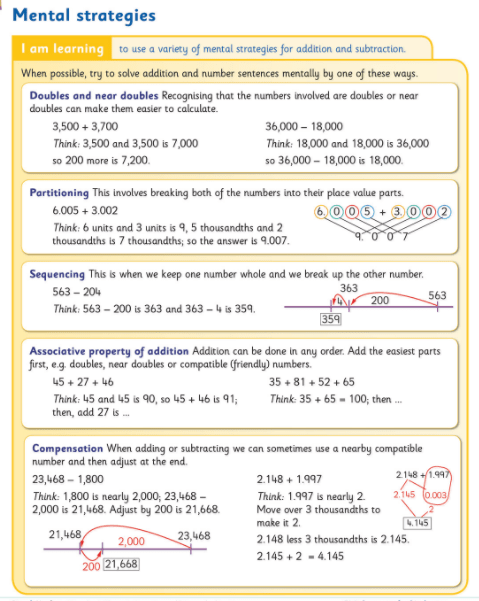Thus, one of the main purposes of the Addition and Subtraction chapters in Operation Maths is to extend the range of mental calculation strategies the children have and to enable them to apply the strategies to numbers of greater complexity i.e. for the children to become efficient and flexible, as well as accurate. As the same calculation can often be done mentally in many different ways, the children have to develop their decision-making skills so as to be in a position to decide what is the most efficient strategy to use in each situation.

It is worth noting that the page from Operation Maths 6 pictured above serves as a synopsis to remind the children of all the strategies they explored individually in the previous Operation Maths books. That said, if the sixth class children are new to Operation Maths and have never encountered these strategies before, they may need to progress at a much slower pace than those who have been using the programme previously, or who may have encountered these strategies, for example a class who used Number Talks. As mentioned in a previous post, the Operation Maths mental strategies listed below are very similar to, and in some cases identical to, those used in Number Talks (if different terminology from Operation Maths is used in Number Talks, the Number Talks terminology is given in brackets).

• Doubles and near doubles
• Number bonds of 10, 100 and 1,000 (Making tens)
• Friendly or Compatible numbers (benchmark/friendly numbers)
• Partitioning (breaking each number into its place value parts)
• Compensation
• Subtraction as take-away (removal/deducation)
• Constant difference subtraction (see below)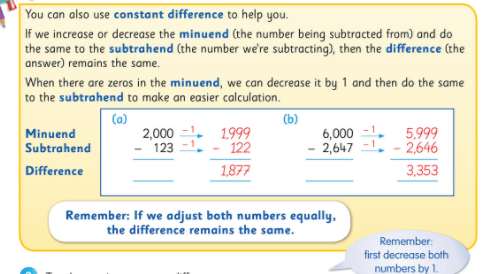Operation Maths also places particular emphasis on the development of estimation skills for number and introduces and develops specific estimation strategies as the books progress. Again, the emphasis is on the children contrasting and comparing these strategies and choosing the most efficient strategy each time. To find out more about some of the estimation strategies, read this post.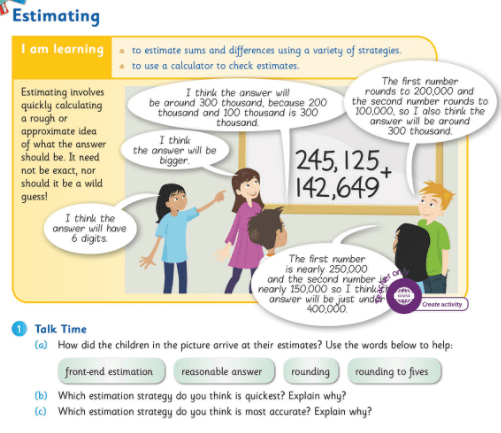Therefore, ask the children, as often as possible when meeting new calculations, can they do it mentally, and how, so that they become increasingly aware of a range of mental calculation skills and approaches. In this way the children will also be developing their decision-making skills, so as to be in a position to decide the most efficient strategy/approach to use.

### Problem-solving strategies

One of the main aims of Operation Maths 3-6 was to introduce both teachers and pupils to a logical problem solving approach (i.e. RUCSAC) , complemented by specific visual problem solving strategies which develop in complexity as the child progresses through the senior classes.

A key step in the RUCSAC problem-solving approach is the ability to read a word problem meaningfully, and highlight the specific operational language or vocabulary. This is reinforced with activities in the Discovery Book (see below) where the children colour-code the specific phrases and then transfer them to their Operations Vocabulary page towards the end of their Discovery Book for future reference.You will notice that the problems have no numbers to distract the children, so that they can just focus on the language of the problems and the operations that may be inferred by the context of the story. These type of “numberless word problems” are being used more and more by practitioners in order to deepen children’s understanding of the concepts involved.

Another key step in the RUCSAC approach is the ability to create to show what you know, where the child makes a representation of the word problem in another form. Bar models are ideal for use with operational word problems. Introduced initially in Operation Maths 3, the use of bar models is developed through Operation Maths 3-6 to include bar models suited to other types of word problems.

Empty number lines can also be used to represent addition and subtraction problems (see below). In the senior books, the children will use both strategies to represent word problems and compare and contrast the two strategies. Ultimately, it is hoped that the children will use the strategy that they are most comfortable with. For more information on problem-solving strategies please consult the guide to problem-solving strategies across the scheme in the introduction to your Teachers Resource Book (TRB) or read on here.### Communicating and expressing thinking

Being able to explain your mathematical thinking is a very powerful tool, and one that can greatly aid the learning and understanding of both the speaker and the listener(s). Encourage the children to verbalise how they did their calculations (mental or written) to provide you with a window on their thinking. When talking about decimal numbers, encourage children to use fractional language as opposed to decimal language, i.e. ‘6 hundredths plus 4 hundredths is ten hundredths’ etc.Another way to communicate and express thinking is via jottings. These are informal diagrams that both show and support thinking, and when used as a part-mental approach, serve as an intermediate stage between concrete materials and the abstract calculation. Their use should be encouraged as much as possible (e.g. “use jottings to show me your thinking”) until the child is confident enough to do the whole calculation mentally or using a traditional written form. The main jottings used in Operation Maths are empty number lines (pictured above) and branching (pictured below) to show part–whole relationships and/or explore compensation.